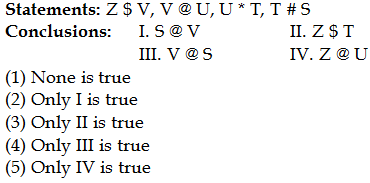## PREPARATORY QUESTIONS

### EQUAL-INEQUAL

#### PREPARATORY SET-36 (HARD)

Direction (Qs.1 to 5): In the following questions, the symbols \$, @, #, & and * are used with the following meaning as illustrated below.

‘A \$ B’ means ‘A is not smaller than B’

‘A \$ B’ means ‘A is not smaller than B’

‘A # B’ means ‘A is neither greater than nor equal to B’

‘A & B’ means ‘A is neither greater than nor smaller than B’

‘A * B’ means ‘A is not greater than B’

Now in each of the following questions assuming the given statement to be true, find which of the conclusions given below them is/are definitely true and give your answer accordingly.

Question No : 1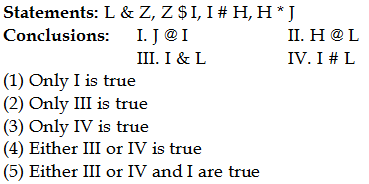Question No : 2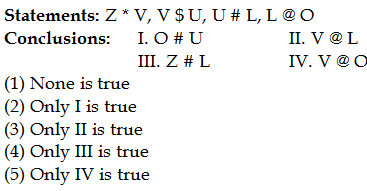Question No : 3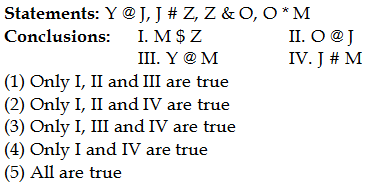Question No : 4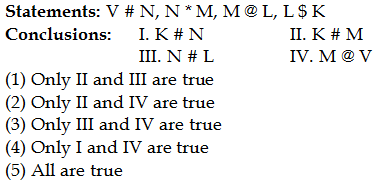Question No : 5